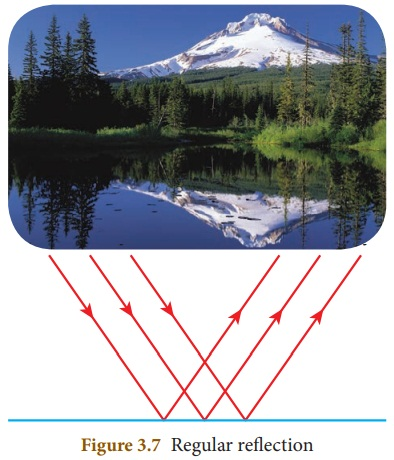Home | | Science 8th Std | Types of Reflection

# Types of Reflection

Based on the nature of the surface, reflection can be classified into two types namely, regular reflection and irregular reflection.

Types of Reflection

We have learnt that not all bodies can reflect light rays. The amount of reflection of light depends on the nature of the reflecting surface of the body. Based on the nature of the surface, reflection can be classified into two types namely, regular reflection and irregular reflection.

1. Regular reflection

When a beam of light (collection of parallel rays) falls on a smooth surface, it gets reflected. After reflection, the reflected rays will be parallel to each other. Here, the angle of incidence and the angle of reflection of each ray will be equal. Hence, the law of reflection is obeyed in this case and thus a clear image is formed. This reflection is called ‘regular reflection’ or ‘specular reflection’. Example: Reflection of light by a plane mirror and reflection of light from the surface of still water.2. Irregular reflection

In the case of a body having a rough or irregular surface, each region of the surface is inclined at different angles. When light falls on such a surface, the light rays are reflected at different angles. In this case, the angle of incidence and the angle of reflection of each ray are not equal. Hence, the law of reflection is not obeyed in this case and thus the image is not clear. Such a reflection is called ‘irregular reflection’ or ‘diffused reflection’. Example: Reflection of light from a wall.Tags : Light | Chapter 3 | 8th Science , 8th Science : Chapter 3 : Light
Study Material, Lecturing Notes, Assignment, Reference, Wiki description explanation, brief detail
8th Science : Chapter 3 : Light : Types of Reflection | Light | Chapter 3 | 8th Science